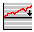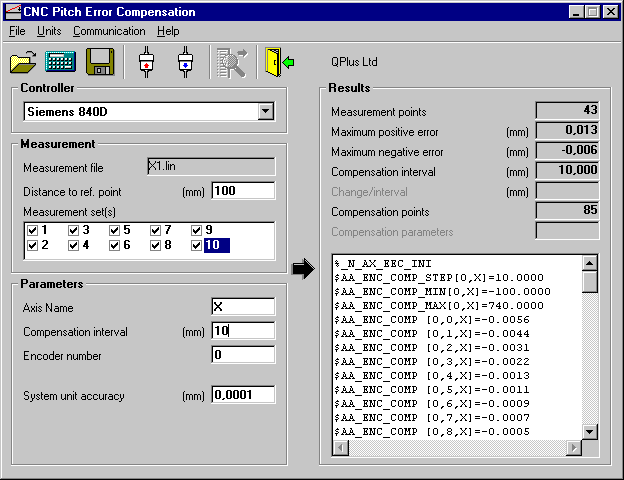## CNCcomp - Siemens 840DCNCcomp

#### Measurement File Options

Measurement file options are the same for all the controller types. Reference point is the distance between machine tool axes reference point and the start point of the measurement. If measurement file includes several measurement runs (sets) they all are listed in a Measurement set(s) listbox. It is possible to select just one or several sets for the compensation. If more than one set is selected the average of all selected measurement sets are used for the compensation.

#### Parameters Options

Axes Name
Axes name is used as given in the compensation file.

Compensation interval
Compensation interval defines how many compensation points are calculated. There is no need to use more compensation points then the measurement points because the controller calculates the compensation points between the measurement points automatically.

Encoder number
It is possible to use different compensations for different encoders. The encoder number defines which encoder is used.

System unit accuracy
System unit accuracy defines how many decimals are shown on the parameter file.

#### Calculation

The calculation is done by pressing the CALCULATE toolbar button.

#### Results

Calculation results are shown on the right side of the form. The actual compensation file format is shown on the lower right corner of the window.

#### Saving the compensation parameter file

Compensation parameter file is saved by pressing the SAVE toolbar button after which the save file dialog is opened.

#### Sample screen